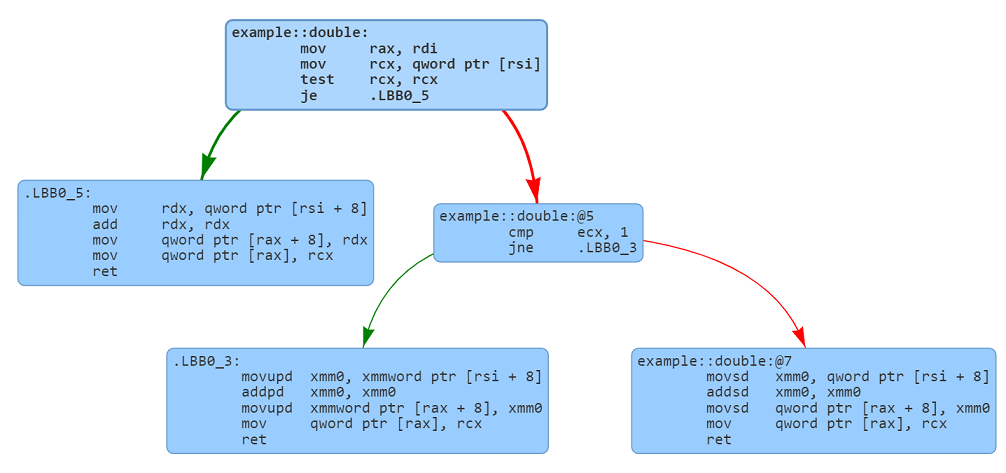# Rust enum-match code generation

We are beginning a series to understand the assembly code generated from Rust. These articles will help us better understand the tradeoffs involved in using different Rust primitives.

## Matching an enum and associated fields

Enums in Rust are discriminated unions that can save one of multiple variants. The enum discriminator identifies the current interpretation of the discriminated union.

The following code shows a simple enum in Rust that represents a generalized `Number` that can be an `Integer`, a `Float` or `Complex`. Here `Number` is a container that can store a 64-bit integer (`i64`), a 64-bit floating point number (`f64`) or a complex number (stored in a struct with two `f64` fields).

The code following the enum declaration, declares a function `double` that takes a `Number` parameter and return a `Number` that doubles the fields of whatever type of `Number` is found in the enum. The match statement in Rust is used to pattern match the contents and return the appropriate variant.

``````pub enum Number {
Integer(i64),
Float(f64),
Complex { real: f64, imaginary: f64 },
}

pub fn double(num: Number) -> Number {
match num {
Number::Integer(n) => Number::Integer(n + n),
Number::Float(n) => Number::Float(n + n),
Number::Complex { real, imaginary } => Number::Complex {
real: real + real,
imaginary: imaginary + imaginary,
},
}
}
``````

## Memory layout of a Rust enum

Before we proceed any further, let's look at the enum organization in memory. The size of the enum depends upon the largest variant. This this example a `Number::Complex` requires two 64-bit floats. The total memory needed for the variant is 16 bytes. The size of the enum is 24 bytes. The extra 8 bytes are used to store a 64-bit discriminator that is used to identify the variant currently saved in the enum.

Byte offset`Integer``Float``Complex`
0DiscriminatorDiscriminatorDiscriminator
8`i64``f64``f64`
16`f64`

Note: A 64-bit discriminator might seem wasteful here. Due to padding rules, a smaller discriminator would not have saved any memory. Rust does switch to a smaller discriminator when reducing the size permits addition of smaller fields.

## Deep dive into the generated code

The following graph shows the overall structure of the generated assembly. The top box and middle-right boxes check the discriminator and then invoke the appropriate variant handling code (the three leaf boxes).We have annotated the assembly code to aid in the understanding of the code. The generated code looks at the discriminator and then access the fields corresponding to selected variants. The code then doubles the individual fields associated with the variant. The enum with doubled values is returned from the function. The function also copies the discriminator field to enum that is being returned.

``````example::double:
mov     rax, rdi                    ; rax now contains the address to the return value
mov     rcx, qword ptr [rsi]        ; Extract the union discriminator
test    rcx, rcx                    ; Check if the discriminator is 0 (Number::Integer)
je      .LBB0_5                     ; Jump if the discriminator is 0.
cmp     ecx, 1                      ; Check if the discriminator is 1 (Number::Float).
jne     .LBB0_3                     ; Jump if discriminator is 2 (Number::Complex)

; Number::Float match processing (discriminator is 1)
movsd   xmm0, qword ptr [rsi + 8]   ; Move the floating point number in xmm0
addsd   xmm0, xmm0                  ; Double the number
movsd   qword ptr [rax + 8], xmm0   ; Save in value in the return value
mov     ecx, 1                      ; Set the discriminator in ecx (lower 32 bits of rcx)
mov     qword ptr [rax], rcx        ; Copy the discriminator into the return value

.LBB0_5:
; Number::Integer match processing (discriminator is 0)
mov     rcx, qword ptr [rsi + 8]    ; Move the integer
add     rcx, rcx                    ; Double the number
mov     qword ptr [rax + 8], rcx    ; Write the number to the return value
xor     ecx, ecx                    ; Set the discriminator to 0 (Number::Integer)
mov     qword ptr [rax], rcx        ; Write the discriminator to the return value
ret

.LBB0_3:
; Number::Complex match processing (discriminator is 2)
movsd   xmm0, qword ptr [rsi + 8]   ; Read the real part
movsd   xmm1, qword ptr [rsi + 16]  ; Read the imaginary part
addsd   xmm0, xmm0                  ; Double the real part
addsd   xmm1, xmm1                  ; Double the imaginary part
movsd   qword ptr [rax + 8], xmm0   ; Update the real part of the return value
movsd   qword ptr [rax + 16], xmm1  ; Update the imaginary part of the return value
mov     ecx, 2                      ; Set the discriminator to 2 (Number::Complex)
mov     qword ptr [rax], rcx        ; Set the discriminator in the return value
ret
``````